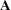BY Statement
BY variables ;

You can specify a BY statement with PROC ACECLUS to obtain separate analyses on observations in groups that are defined by the BY variables. When a BY statement appears, the procedure expects the input data set to be sorted in order of the BY variables. If you specify more than one BY statement, only the last one specified is used.

If your input data set is not sorted in ascending order, use one of the following alternatives:

• Sort the data by using the SORT procedure with a similar BY statement.

• Specify the NOTSORTED or DESCENDING option in the BY statement for the ACECLUS procedure. The NOTSORTED option does not mean that the data are unsorted but rather that the data are arranged in groups (according to values of the BY variables) and that these groups are not necessarily in alphabetical or increasing numeric order.

• Create an index on the BY variables by using the DATASETS procedure (in Base SAS software).

If you specify the INITIAL=INPUT= option and the INITIAL=INPUT= data set does not contain any of the BY variables, the entire INITIAL=INPUT= data set provides the initial value for the matrixfor each BY group in the DATA= data set.

If the INITIAL=INPUT= data set contains some but not all of the BY variables, or if some BY variables do not have the same type or length in the INITIAL=INPUT= data set as in the DATA= data set, then PROC ACECLUS displays an error message and stops.

If all the BY variables appear in the INITIAL=INPUT= data set with the same type and length as in the DATA= data set, then each BY group in the INITIAL=INPUT= data set provides the initial value forfor the corresponding BY group in the DATA= data set. All BY groups in the DATA= data set must also appear in the INITIAL= INPUT= data set. The BY groups in the INITIAL=INPUT= data set must be in the same order as in the DATA= data set. If you specify NOTSORTED in the BY statement, identical BY groups must occur in the same order in both data sets. If you do not specify NOTSORTED, some BY groups can appear in the INITIAL= INPUT= data set, but not in the DATA= data set; such BY groups are not used in the analysis.